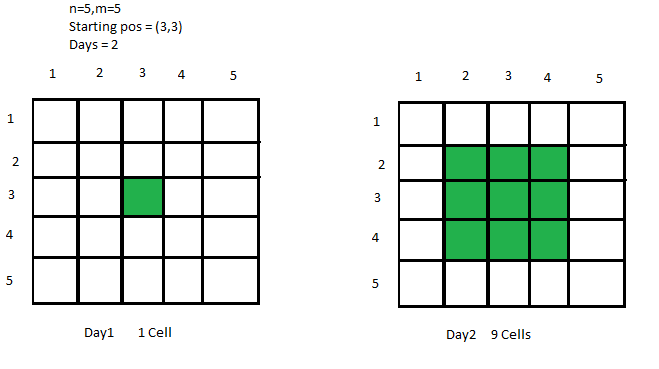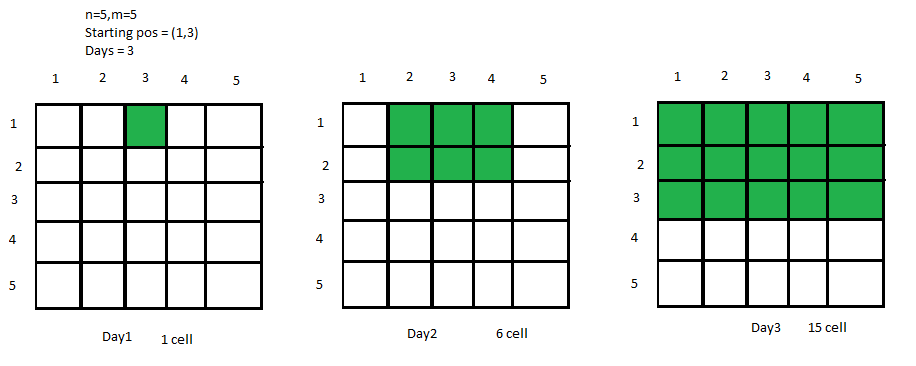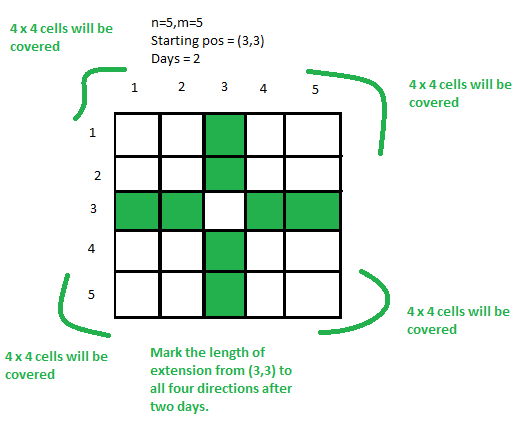Total number of cells covered in a matrix after D days

• Last Updated : 05 May, 2021

Given an N * M matrix and a starting position (X, Y) of a virus, the task is to find out the number of covered cells after D days, if the virus spreads from its current cell to its adjacent cells every day.Examples:

Input: N = 5, M = 5, X = 1, Y = 3, D = 3Output: 15
Explanation:
We can clearly see from the picture that 15 cells are covered after 3 days.
Input: N = 10, M = 10, X = 7, Y = 8, D = 4
Output: 42
Explanation:
On making an N * M matrix and filling the adjacent cells for 4 days we will get 42 covered cells.

Approach:
To solve the problem mentioned above we have to observe clearly that from a starting cell, we just need to find out the extension of cells towards top, right, bottom and left after D days. Then calculate the total cells inside every quadrilateral of cells formed and add them all.
Therefore, the total answer will be the sum of all cells of quadrilaterals after D days + the total cells that are along the top, right, down, left, and 1 (for Starting cell) keeping in consideration the boundaries of the quadrilateral.
Below is the condition for extension in all four directions:

Extension upto Top -> min(D, X-1)
Extension upto Down -> min(D, N-X)
Extension upto Left -> min(D, Y-1)
Extension upto Right -> min(D, M-Y)

Look at the image below for clear understanding:Now multiply Top * Left, Top * Right, Down * Left, Down * Right and add all of them and also add the total cells along the line of 4 directions. We also add 1(for starting cell) to get the resultant cells.
Below is the implementation of above approach:

Javascript


Output:
42

Time Complexity: O(1)

My Personal Notes arrow_drop_up